Quartiles, deciles and percentile

 Page 5/6 Date 03.05.2017 Size 341.52 Kb. #18702

Quartiles, deciles and percentile

For Ungrouped data

• Quartiles: divide the distribution into four group Q1 , Q2 , Q3

Smallest data Q1 Q2 Q3 Largest data

25% 25% 25% 25%

Median

• arrange the data in order

• Find location of quartiles,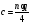where ; n = total number of values

q =quartile

1. If c is not whole number, round up to the next whole number

2. If c is a whole number, take average of cth and (c+1)th

Example:

1. The weights in pounds in the data set. Find Q1 , Q2 , Q3.

16 18 22 19 3 21 17 20

1. The test score in the data set. Find Q1 , Q2 , Q3.

42 35 28 12 47 50 49

• Deciles: divide the distribution into 10 groups

Smallest data D1 D2 D3 D4 D5 D6 D7 D8 D9 Largest data

10% 10% 10% 10% 10% 10% 10% 10% 10%

Median

• arrange the data in order

• Find location of quartiles,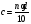where ; n = total number of values

d =decile

1. If c is not whole number, round up to the next whole number

2. If c is a whole number, take average of cth and (c+1)th

Example:

1. (from previous example) Find D5.

16 18 22 19 3 21 17 20

1. (from previous example)Find D7.

42 35 28 12 47 50 49

Smallest data P1 P2 P3 P97 P98 P99 Largest data

10% 10% 10% 10% 10% 10% 10% 10% 10%

D1 , D2, D3, … , D9 correspond to P10 , P20, P30, … , P90

Q1 , Q2 , Q3 correspond to P25 , P50, P75

Median = Q2 = D5 = P50

• arrange the data in order

• Find location of quartiles,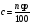where ; n = total number of values

p =percentile

1. If c is not whole number, round up to the next whole number

2. If c is a whole number, take average of cth and (c+1)th

Example:

1. (from previous example) Find P33.

16 18 22 19 3 21 17 20

1. (from previous example)Find P60.

42 35 28 12 47 50 49

Finding percentile corresponding to given value, X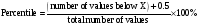Example of data set : 1 1 3 4 5

Find percentile for 4.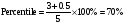P70 = 4

Example:

1. (from previous example)Find the percentile rank for each test score in the data set.

42 35 28 12 47 50 49

(Data value 47 = P64 but previously when we want to find P60 the data value is 47b too. So actually P60 closer to P64 which is data value 47)

For Grouped Data

METHOD 1: (USE PERCENTILE GRAPH)

x-axis: class boundaries

y-axis: relative cumulative frequency (percentage)

Cumulative relative frequency (%) =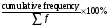Graph:

1. percentile graph

Relative cumulative frequency (%)

100

25 P25

1. Ogive using relative frequency (iii) Ogive

Relative cumulative frequency Cumulative Frequency

1.0 75

0.25 P25 18.75 P25

25% x 75 =18.75

METHOD 2: (USE FORMULA)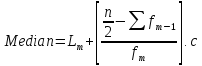Example:

This distribution represents the data for weights of fifth-grade boys.

 Weights (pounds) frequency 52.5 – 55.5 9 55.5 – 58.5 12 58.5 – 61.5 17 61.5 – 64.5 22 64.5 – 67.5 15

1. Find the approximate weights corresponding to each percentile given by constructing a percentile graph.

(i) Q1 (ii) D8 (iii) Median (iv) P95

1. Find the approximate percentile ranks of the following weights.

(i) 57 pounds (ii) 64 pounds (iii) 62 pounds (iv) 59 pounds

1. Find P63 by using the formula.

EXERCISE CHAPTER 3

1. What type of sampling is being employed if a country is divided into economic classes and a sample is chosen from each class to be surveyed?

2. Given a set of data 5,2,8,14,10,5,7,10,m, n where =7 and mode = 5. Find the possible values of m and n. (ans: m=5, n=4 or m =4 , n =5)

3. Find the value that corresponds to the 30th percentile of the following data set:

78 82 86 88 92 97 (ans: P30 =82)

1. Given the variance of the set of 8 data x1 , x2, x3, … , x8 is 5.67. If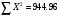, find the mean of the data. (ans: 11.09)

2. Find Q3 for the given data set : 18,22,50,15,13,6,5,12 (ans: 20)

3. The number of credits in business courses that eight applicants took is 9, 12, 15, 27, 33, p, 63, 72. Given the value that corresponds to the 75th percentile is 54, find p. (ans: 45)

4. The mean of 5, 10, 26, 30, 45, 32, x, y is 25 where x and y are constants. If x = 16, find the median. (ans: 28)

1. Construct a frequency distribution by using 7 classes (use 3 as lower limit of the first class)

2. Find the mean, mode and standard deviation. (ans: 28.15 , 31.3 , 14.63)

3. Draw an ogive by using relative frequency and estimate the median from the graph.

EXERCISE

1. In four successive history tests, a student received grades of 45, 73, 77 and 86. Which of the following conclusions can be obtained from these figures by descriptive method and inferential method? Explain your answer.

1. Only one of the grades exceeds 85.

2. The student’s grades increased from each test to the next.

3. The student must have studied harder for each successive test.

4. The difference between the highest and the lowest grade is 41.

1. State whether the following are nominal, ordinal, interval or ratio data.

1. A statistics test which a student took was easy, difficult or very difficult and these alternatives are coded 1, 2 and 3.

2. The temperature if different kilns at the factory.

3. The bottles on a Chemistry laboratory shelf are numbered 1,2,3 and 4 representing sulfuric acid, hydrochloric acid, nitric acid and sodium hydroxide.

4. The race of the students in university campus.

5. The normal operating temperature of a car engine.

6. Classification of students using an academic program.

7. Speaker of a seminar rated as excellent, good, average or poor.

8. Number of hour’s parents spends with their children per day.

EXERCISE

1. In four successive history tests, a student received grades of 45, 73, 77 and 86. Which of the following conclusions can be obtained from these figures by descriptive method and inferential method? Explain your answer.

1. Only one of the grades exceeds 85.

2. The student’s grades increased from each test to the next.

3. The student must have studied harder for each successive test.

4. The difference between the highest and the lowest grade is 41.

1. State whether the following are nominal, ordinal, interval or ratio data.

1. A statistics test which a student took was easy, difficult or very difficult and these alternatives are coded 1, 2 and 3.

2. The temperature if different kilns at the factory.

3. The bottles on a Chemistry laboratory shelf are numbered 1,2,3 and 4 representing sulfuric acid, hydrochloric acid, nitric acid and sodium hydroxide.

4. The race of the students in university campus.

5. The normal operating temperature of a car engine.

6. Classification of students using an academic program.

7. Speaker of a seminar rated as excellent, good, average or poor.

8. Number of hour’s parents spends with their children per day.